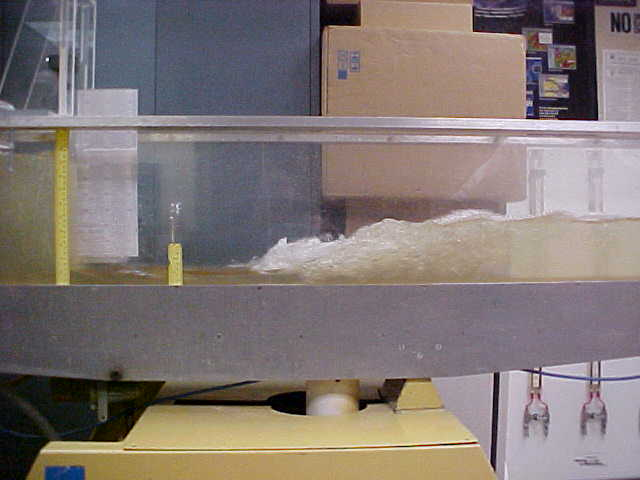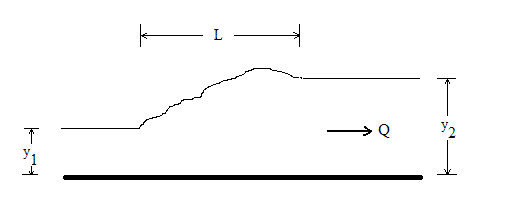Hydraulic Jump in Horizontal Rectangular Channel

Enter discharge, channel width, upstream depth. Compute downstream depth, Froude numbers, depth ratio, velocities, hydraulic jump length and energy loss.

Register to enable "Calculate" button.

 Channel width, B: Width in cm Width in meters Width in inches Width in feet Discharge, Q: Discharge in m3/s Discharge in m3/min Discharge in liter/s Discharge in liter/min Discharge in ft3/s Discharge in ft3/min Discharge in gal/min (US) Discharge in gal/hr (US) Discharge in gal/day (US) Discharge in MGD Upstream depth, Y1: Depth and Loss in cm Depth and Loss in meters Depth and Loss in inches Depth and Loss in feet Downstream depth, Y2: Jump length in cm Jump length in meters Jump length in inches Jump length in feet Depth ratio, Y2/Y1: Velocity in m/s Velocity in ft/s Jump length, L: Head loss, h: © 2014 LMNO Engineering, Upstream Froude, F1: Research, and Software, Ltd. Downstream Froude, F2: http://www.LMNOeng.com Upstream velocity, V1: Downstream velocity, V2:

Hydraulic jump calculation units: cm=centimeter, ft=foot, gal=US gallon, hr=hour, m=meter, MGD=Million Gallons (US) per Day, min=minute, s=second.Photograph from Ohio University's Fluid Mechanics Laboratory. Athens, Ohio USA

Hydraulic Jump Equations

Equations for hydraulic jump in horizontal rectangular channel (Chaudhry, 1993; Chow, 1959):

Q = V1 Y1 B = V2 Y2 B      F1 = V1 / (g Y1)0.5      F2 = V2 / (g Y2)0.5

Y2/Y1 = 0.5 [(1+8F12 )0.5 - 1]      L = 220 Y1 tanh[(F1-1)/22]        h = (Y2-Y1)3 /( 4 Y1 Y2)

where (subscript 1 indicates upstream of jump; subscript 2 indicates downstream of jump):
B=Channel width (m), F=Froude number (dimension-less), g=acceleration due to gravity (9.8066 m/s2), h=Head loss (m), L=Length of jump (m), Q=Discharge (m3/s), tanh=Hyperbolic tangent trigonometric function, V=Velocity (m/s), Y=Water depth (m)

What is a Hydraulic Jump?

A hydraulic jump occurs when the upstream flow is supercritical (F>1). To have a jump, there must be a flow impediment downstream. The downstream impediment could be a weir, a bridge abutment, a dam, or simply channel friction. Water depth increases during a hydraulic jump and energy is dissipated as turbulence. Often, engineers will purposely install impediments in channels in order to force jumps to occur. Mixing of coagulant chemicals in water treatment plants is often aided by hydraulic jumps. Concrete blocks may be installed in a channel downstream of a spillway in order to force a jump to occur thereby reducing the velocity and energy of the water. Flow will go from supercritical (F>1) to subcritical (F<1) over a jump.

According to Chow (1959), a strong jump occurs when F1>9, a steady jump occurs when 4.5<F1<9, an oscillating jump occurs when 2.5<F1<4.5, a weak jump occurs when 1.7<F1<2.5, and an undular jump occurs when 1<F1<1.7. According to Chaudhry (1993), the best jumps occur when 4.5<F1<9.

Messages
"Need B>0."  Channel width must be a positive number.
"Need Q>0."  Discharge must be positive.
"Need Y1>0."  Upstream depth must be positive.
"Need F1 >1."  Upstream flow must be supercritical.

References
Chaudhry, M. H. 1993. Open Channel Flow. Prentice-Hall, Inc.

Chow, V. T. 1959. Open Channel Hydraulics. McGraw-Hill, Inc.